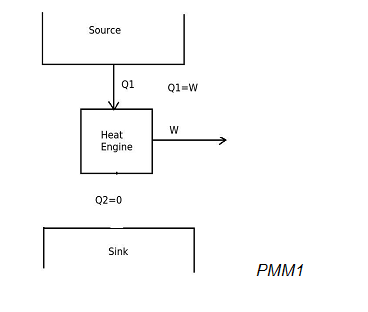# If a heat engine produces net work output by exchanging heat with only one reservoir, then the heat engine will be,

## If a heat engine produces net work output by exchanging heat with only one reservoir, then the heat engine will be,

a. perpetual motion machine of first kind (PMM1)
b. perpetual motion machine of second kind (PMM2)
c. perpetual motion machine of third kind (PMM3)
d. none of the above

Correct Answer : b. perpetual motion machine of second kind (PMM2)

Explanation :

When a heat engine produces net work output by exchanging heat with only one reservoir, then it will be represented as,In the above diagram, the heat engine is utilizing input heat energy and converting it completely into work output. According to the Kelvin-Planck statement of second law of thermodynamics, it is impossible to produce a heat engine, whose sole effect is to absorb energy in the form of heat from a heat source and produce an equal amount of work. This is due to that some heat has to be rejected to sink. Therefore this heat engine violates the Kelvin-Planck statement of second law of thermodynamics. Hence it will be a perpetual motion machine of second kind (PMM2). PMM2 is impossible to produce.